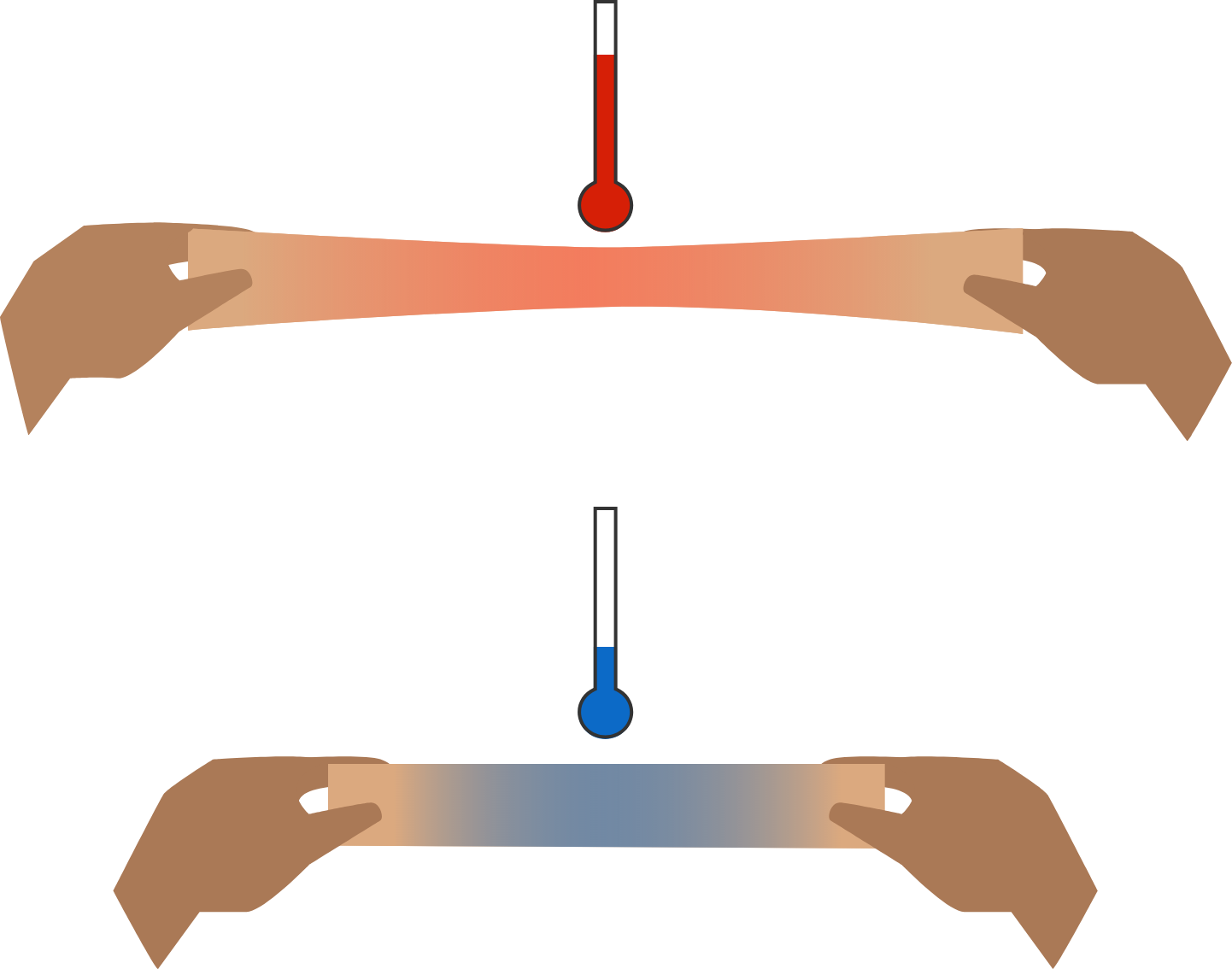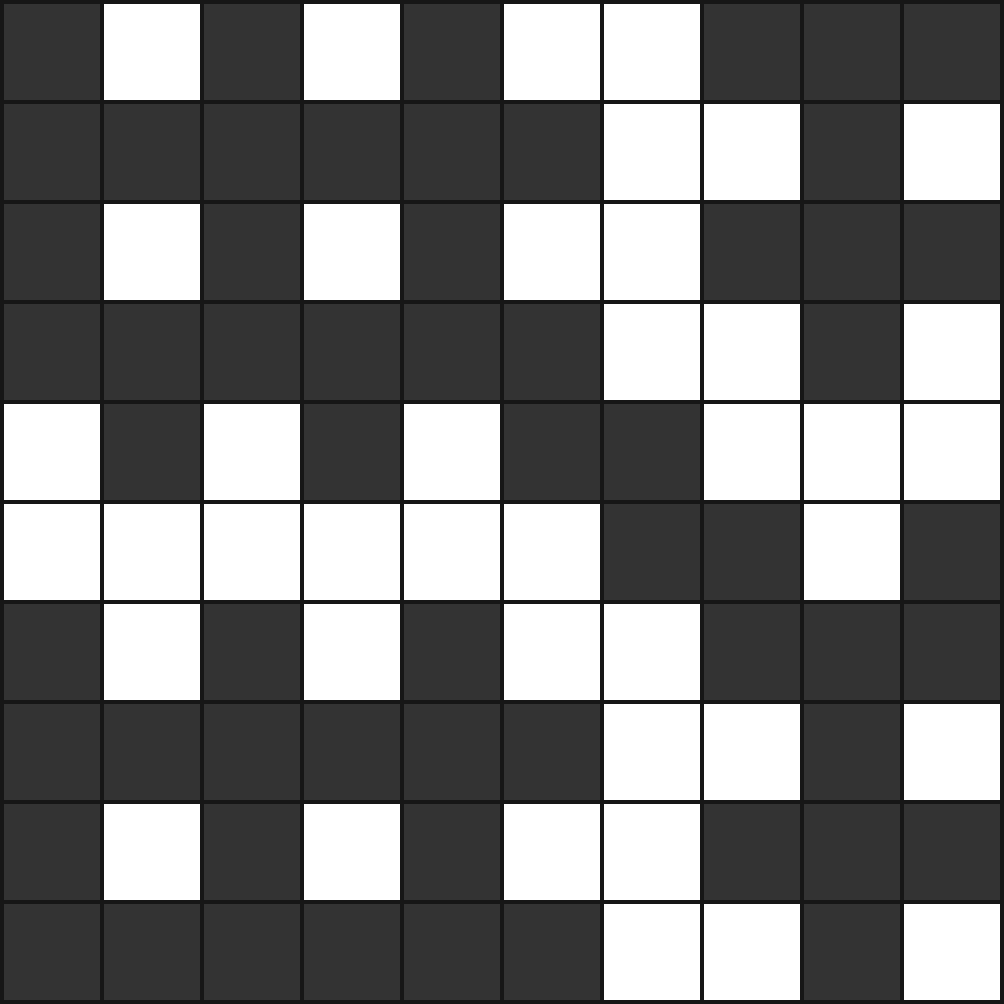# Problems of the Week

Contribute a problem

Rubber heats up when it expands and cools when it contracts. What is the physical cause for this strange effect?

For example, if you hold a rubber band in both hands and expand it very quickly, you will find that it heats up significantly. After cooling it to room temperature and then quickly shrinking it back to its original length, you'll notice that it cools even further.If you pick a number $x$ uniformly at random on the interval $[0,1],$ what is the probability that $\left\lfloor \log _{2}\frac{1}{x}\right\rfloor$ is odd, to 3 significant figures?

Note: $\lfloor \cdot \rfloor$ is the floor function.

Starting with a number, the following is called the Collatz rule:

If the number is odd, multiply by 3 and add 1.

If the number is even, divide by 2.

The Collatz conjecture suggests that when you keep doing this, you will always reach 1 eventually.

For example, if you start with 7, you reach $1$ in 16 steps: $7 \to 22 \to 11 \to 34 \to 17 \to 52 \to 26 \to 13 \to 40 \to 20 \to 10 \to 5 \to 16 \to 8 \to 4 \to 2 \to 1.$ However, 7 is not the only number that requires 16 steps. What is the total number of positive integers which require exactly 16 steps to reach 1 for the first time?

Note: This problem is intended to have a coding solution.

The number 24 has a special property: all of its digits appear at the end of its cube, 13824, in the same order.

How many positive integers less than 1000 (including the number 24) have this property?

How many different colorings of a $10 \times 10$ square grid are there such that the following 2 conditions are met?

1. Each square must be either black or white.
2. Each $2 \times 2$ square in the grid must contain an odd number of $1 \times 1$ black squares.An example of how the grid can be colored

×